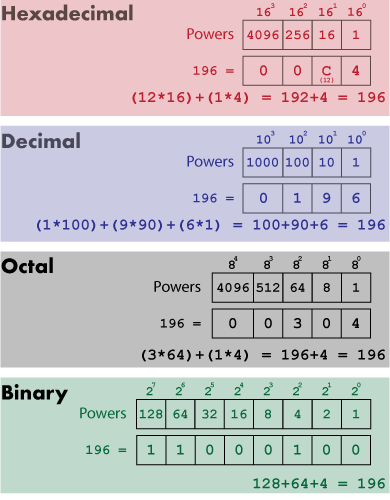# Dreaming of the ternary_out() Function

To generate a string of digits representing a value in a specific power base, such as base 3 (ternary), you need a power table. Using this power table, you can translate any positive integer into a string representation of the number in the given base. Sounds complex. Is complex.

In last week’s Lesson, I presented code that built a powers table for a value. For my ternary insanity, the table contains powers of 3. From this table I can construct a function that outputs a positive integer as a base-3 value, which consists only of digits 0, 1, and 2.

Ideally, I think the ternary counting system would show digits 1, 0, and -1 — or better: +, 0 and -. This base plays well in to three-way evaluations, which was the topic of an older blog post. My point with devising a ternary_out() function is merely to express a value in base 3.

Figure 1 illustrates how base 3 counting works for the value 196.Figure 1. Base 3 counting.

The value 196 is expressed as 21021 in base 3. It works like this:

`(2×34) + (1×33) + (0×32) + (2×31) + (1×30)`

``` (2×81) + (1×27) + (0×9) + (2×6) + (1×1) ```

`162 + 27 + 0 + 6 + 1`

This same type of translation is done for all counting systems. In Figure 2, you see the calculations for value 196 is bases 16, 10, 8, and 2.Figure 2. Counting systems.

For base 3, a similar calculation is made. But first, I decided to craft the code as shown below, creating a skeleton of the ternary_out() function with error checking for input, the powers table, and a dummy string:

### 2020_06_06-Lesson.c

```#include <stdio.h>
#include <stdlib.h>

char *ternary_out(unsigned n)
{
static char tstring;
int powers;
int x;

/* check for overflow */
if( n<0 || n>65535 )
{
fprintf(stderr,"%d is out of range\n",n);
exit(1);
}

/* create the 3 powers table */
powers = 1;
for(x=1;x<12;x++)
{
powers[x] = powers[x-1]*3;
}

/* build the return string */
tstring = '\0';

return(tstring);
}

int main()
{
unsigned t;

/* prompt for input */
printf("Enter a valued: ");
scanf("%d",&t);

printf("%d in ternary is %s\n",t,ternary_out(t));

return(0);
}```

Here’s the unimpressive output:

```Enter a value in ternary: 196 196 in ternary is```

This result is fine because I haven’t yet coded the guts of the function. This issue presents the tallest hurdle — especially because I wasn’t going to rush off to StackOverflow to see how someone else might have done it or how the compiler itself outputs values in hex and octal. No, I had only my brain, a pad of graph paper, and Excel. Yes, I used a spreadsheet to help me refine my algorithm. After all, I prefer the computer to do the math.

Here’s my thought process:

1. Take a value and determine the largest power divisible by that value.
2. Subtract the power (or multiple thereof) from the value.
3. Repeat the process on the remainder as you descend through the power table.

For example, the value 196: It’s less than 35 or 243, so you divide by the next-lowest power of three, 34 or 81:

`196 ÷ 34 = 196 ÷ 81 = 2.419753086419753`

Lop off the change and you 2 for the 34 place digit.

Subtract `2 * 81` from 196 and you get: `196 - 162 = 34`. The process repeats with 34:

`34 ÷ 33 = 34 ÷ 27 = 1.259259259259259`

Minus the change, 1 is the 33 place digit. Then: `34 - 27 = 7`. And . . . you get the idea.

A problem appears with this approach, however, which is why it didn’t work as I coded it and I gave up. Read more about why and see my code for ternary_out() in next week’s Lesson.